﻿ 奇异值分解与深度学习在轴承故障诊断中的应用

# 奇异值分解与深度学习在轴承故障诊断中的应用Application of Singular Value Decomposition and Deep Learning in Bearing Fault Diagnosis

Abstract: Rolling bearings are important parts of mechanical equipment, if not timely detection of failure will cause significant losses. In order to solve this problem, this paper proposes the feature extraction of the waveform by singular value decomposition (SVD), and input the extracted feature into the deep belief network (DBN) for fault diagnosis. Firstly, the input signal is reconstructed and is decomposed by singular value, and then the singular value is taken as the characteristic, which is taken as the input of the deep belief network (DBN) for fault diagnosed. Experiments show: In many experiments, comparison with existing support vector machine (SVM) fault diagnosis method, the accuracy of the proposed method is 98.4%, the variance is 0.42, the diagnostic speed is 0.3 seconds, and the diagnostic accuracy of the existing SVM diagnostic method is 94.7%, the variance is 0.50, and the diagnostic speed is 0.6 second. The method proposed has good accuracy, stability and fastness.

1. 引言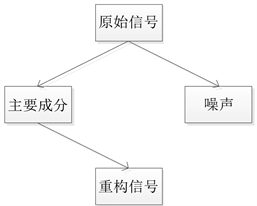(a)(b)

Figure 1. Algorithm structure

2. 背景知识

2.1. SVD的特征提取算法

2.1.1. 奇异值分解原理

$D=\left(\begin{array}{cccc}{x}_{1}& {x}_{2}& \cdots & {x}_{n}\\ {x}_{2}& {x}_{3}& \cdots & {x}_{n+1}\\ ⋮& ⋮& \ddots & ⋮\\ {x}_{m}& {x}_{m+1}& \cdots & {x}_{m+n-1}\end{array}\right)$ (1)

$D=US{V}^{\text{T}}$ (2)

$\begin{array}{l}U=\left[{u}_{1},{u}_{2},\cdots ,{u}_{M}\right]\in {R}^{M×M},\\ V=\left[{v}_{1},{v}_{2},\cdots ,{v}_{N}\right]\in {R}^{N×N},\\ D=\left[\text{diag}\left[{\lambda }_{1},{\lambda }_{2},\cdots ,{\lambda }_{Q}\right],0\right]\text{}\left(Q=\mathrm{min}\left\{M,N\right\}\right)\end{array}$ (3)

$D$ 分解后的值向量为 $\left({\lambda }_{1},{\lambda }_{2},\cdots ,{\lambda }_{Q}\right)$ ，其中 ${\lambda }_{1}\ge {\lambda }_{2}\ge \cdots \ge {\lambda }_{Q}$ 。并将 ${\lambda }_{i}\left(i=1,2,\cdots ,Q\right)$ 的值视为模型特征。

2.1.2. 轴承故障特征提取

2.2. 深度学习方法

RBM是一个层间相互独立并且全连接的条件概率模型。因此想得到每个隐层神经元的状态，可以借助于概率 $P\left(h/v\right)={\prod }_{j=1}^{N}p\left({h}_{j}/v\right)$ ，前提是显层状态已知。这里假设隐藏层单元个数为n。同样，通过隐层状态求导显层状态模式一样，只不过依赖的条件概率为： $P\left(v/h\right)={\prod }_{i=1}^{M}p\left({v}_{i}/h\right)$ ，这里假设显层单元个数为M。在设计RBM时，我们需要设计显示层维数(等于输入数据维度大小)和隐藏层单元数目(提前设定)。假设训练一个RBM模型，可见层单元数目M，偏置为m维列向量，隐藏单元数目n，偏置为n维列向量，这样就需要训练 $\left(M×N+m+n\right)$ 个值。RBM的训练算法采用CD  算法，详细步骤如下：

${h}_{j}=\left\{\begin{array}{cc}1,& p\left({h}_{j}=1\right)\ge u\\ 0,& p\left({h}_{j}=1\right)\le u\end{array}$ (4)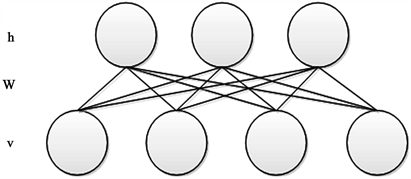Figure 2. Restricted Boltzmann model

3. 奇异值分解与深度学习在轴承故障诊断中的应用

3.1. 数据预处理

3.2. 奇异值分解进行特征提取

$A=\left(\begin{array}{ccc}{x}_{1}& \cdots & {x}_{350}\\ ⋮& \ddots & ⋮\\ {x}_{50}& \cdots & {x}_{400}\end{array}\right)$ (5)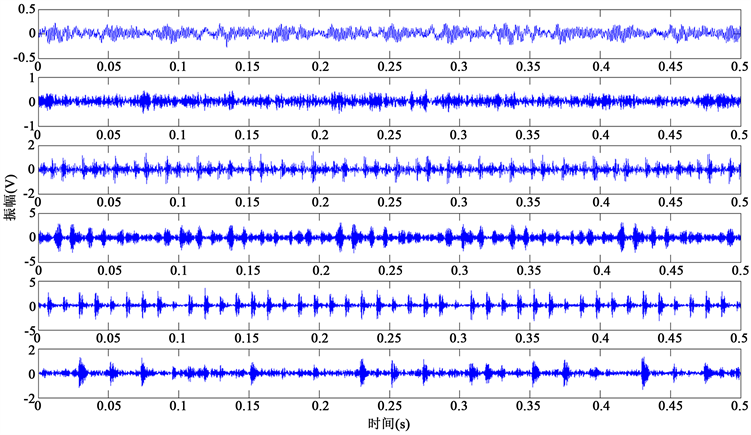Figure 3. Bearing vibration signal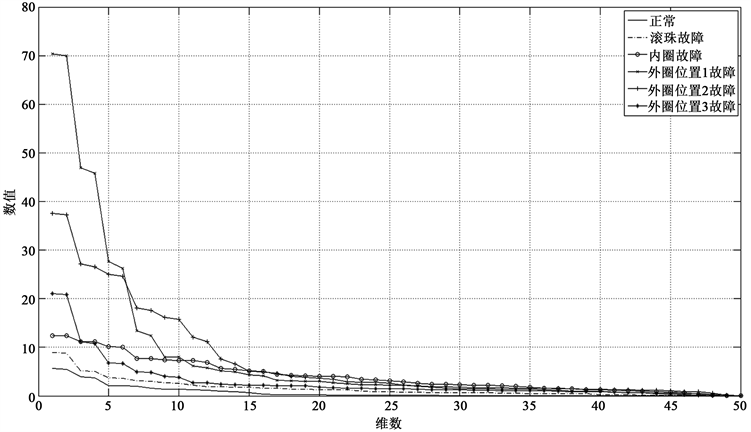Figure 4. Singular value distribution curve

3.3. 模型训练

3.3.1. DBN网络深度

3.3.2. 隐藏层单元个数

Hinton在2012年的研究  中指出，模型的训练过程中，用来表征样本的类别需要的比特数，通常等于一个训练样本施加在参数上的约束项的数量，可以用如下表达式计算：Figure 5. DBN classifier structure(a)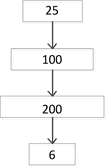(b)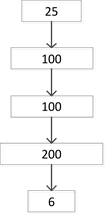(c)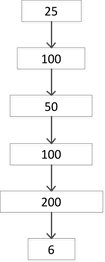(d)

Figure 6. DBN four different depth classifiersTable 1. Recognition accuracy of BDN at different depths

$\mathrm{bits}=-{\mathrm{log}}_{2}x$ (6)

${N}_{p}={N}_{s}×\text{bits}/10$ (7)

3.3.3. 权重初始化、批量选择和全局优化算法选择

${\theta }^{\left(t+1\right)}={\theta }^{\left(t\right)}+\epsilon \left(\frac{1}{B}{\sum }_{{t}^{l}=B×t+1}^{B\left(t+1\right)}\frac{\partial \mathrm{log}P\left({v}^{{t}^{l}}/\theta \right)}{\partial \theta }\right)$ (8)

B为小批量的容量。

3.4. 实验结果分析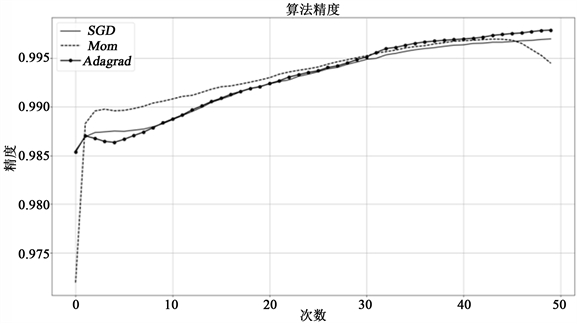Figure 7. Global training accuracy

3.5. 奇异值DBN方法与现有识别方法的比较

4. 结论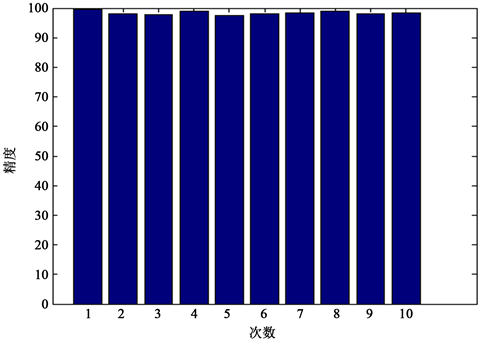Figure 8. 10 experimental results

1) 首先对数据集进行了特征提取，可以滤除掉一部分的噪声，同时结合使用能够更好拟合数据的深度学习方法，所以能够取得更好的精度。

2) 本文应用的深度学习方法需要充足的数据作为支撑，实验数据以及应用数据需要充足，如果没有充足的数据作为支持，效果可能会很不理想。

3) 迁移学习可以进一步解决(2)中涉及的缺点，因此在数据不够充足的情况下可以借助与迁移学习、深度学习结合方法来进行一定的补偿。

NOTES



*通讯作者。

 Kankar, P.K., Sharma, S.C. and Harsha, S.P. (2011) Rolling Element Bearing Fault Diagnosis Using Wavelet Transform. Neurocomputing, 74, 1638-1645.
https://doi.org/10.1016/j.neucom.2011.01.021

 Huang, J., Hu, X. and Yang, F. (2011) Support Vector Machine with Genetic Algorithm for Machinery Fault Diagnosis of High Voltage Circuit Breaker. Measurement, 44, 1018-1027.
https://doi.org/10.1016/j.measurement.2011.02.017

 王欣彦, 王立鹏, 李新. 基于LM-BP神经网络的电机转子裂纹故障诊断[J]. 微特电机, 2015, 43(4): 18-20.

 姜涛, 袁胜发. 基于改进小波神经网络的滚动轴承诊断[J]. 华中农业大学学报, 2014, 33(1): 131-136.

 代荡荡, 王先培, 赵宇, 田猛, 龙嘉川, 朱国威, 张龙飞. 一种改进的奇异值降噪阶次选取方法用于紫外光谱信号去噪的研究[J]. 光谱学与光谱分析, 2016, 36(7): 2139-2143.

 Hinton, G.E., Osindero, S. and Teh, Y.W. (2006) A Fast Learning Algorithm for Deep Belief Nets. Neural Computation, 18, 1527-1554.

 Roux, N.L. and Bengio, Y. (2008) Representational Power of Restricted Boltzmann Machines and Deep Belief Networks. Neural Computation, 20, 1631-1649.
https://doi.org/10.1162/neco.2008.04-07-510

 张春霞, 姬楠楠, 王冠伟. 受限波尔兹曼机[J]. 工程数学学报, 2015, 32(2): 1005-3085.

 马帅, 沈韬, 王瑞琦, 赖华, 余正涛. 基于深层信念网络的太赫兹光谱识别[J]. 光谱学与光谱分析, 2015, 35(12): 3325-3325.

 Hinton, G.E. (2012) A Practical Guide to Training Restricted Boltzmann Machines. In: Neural Networks: Tricks of the Trade, Springer Berlin Heidelberg, 599-619.

 Fischer, A. and Igel, C. (2013) Training Restricted Boltzmann Machines: An Introduction. Pattern Recognition, 47, 25-39.
https://doi.org/10.1016/j.patcog.2013.05.025

 贺昱曜, 李宝奇. 一种组合型的深度学习模型学习率策略[J]. 自动化学报, 2016, 42(6): 953-958.

Top